Miscellaneous (Term 1 and Term 2)

Chapter 13 Class 11 Limits and Derivatives (Term 1 and Term 2)
Serial order wise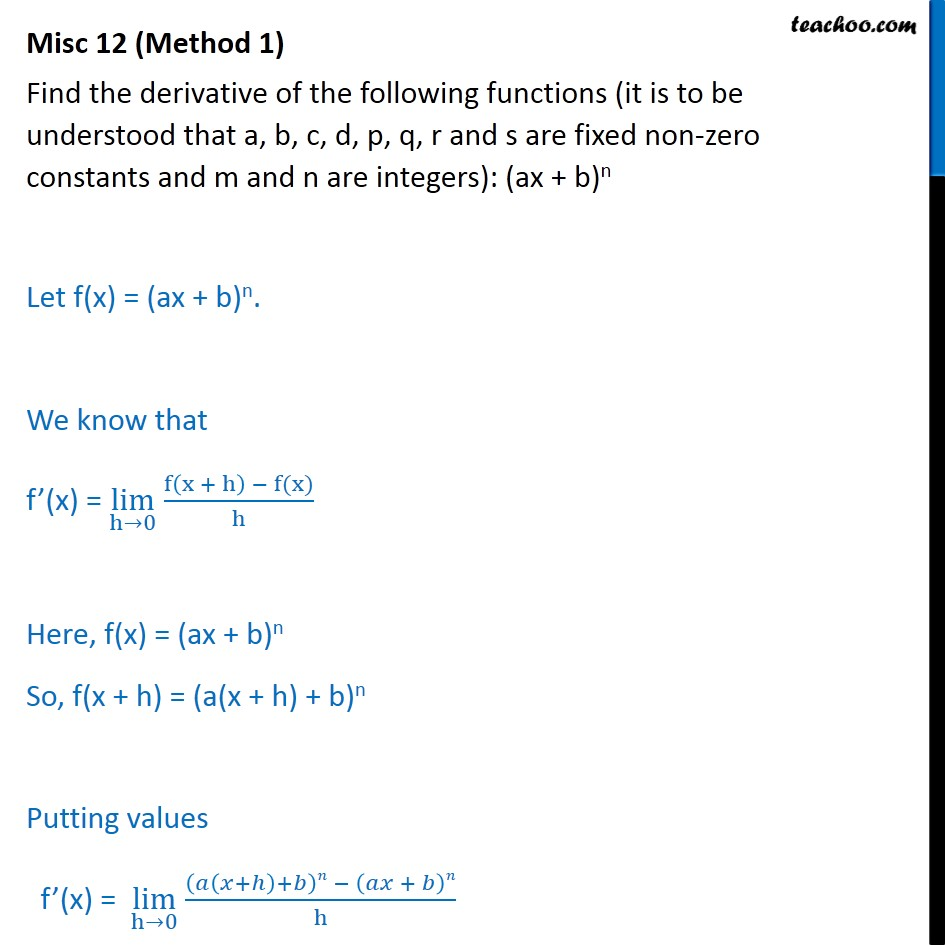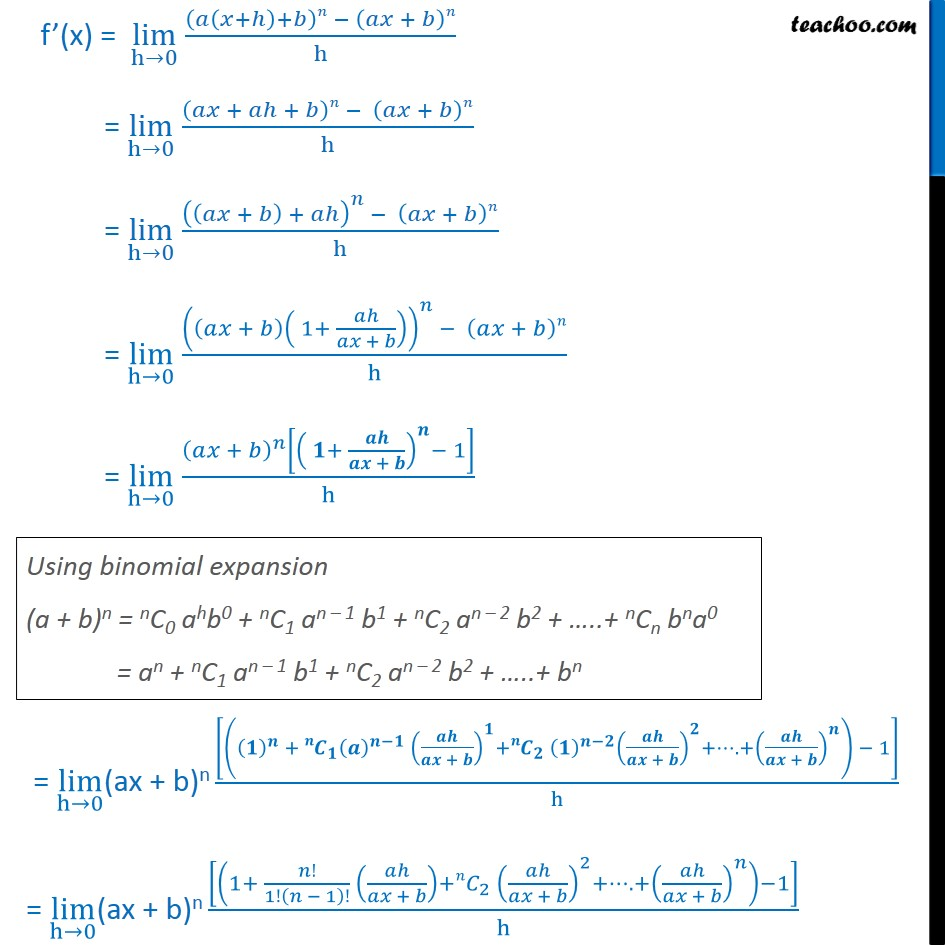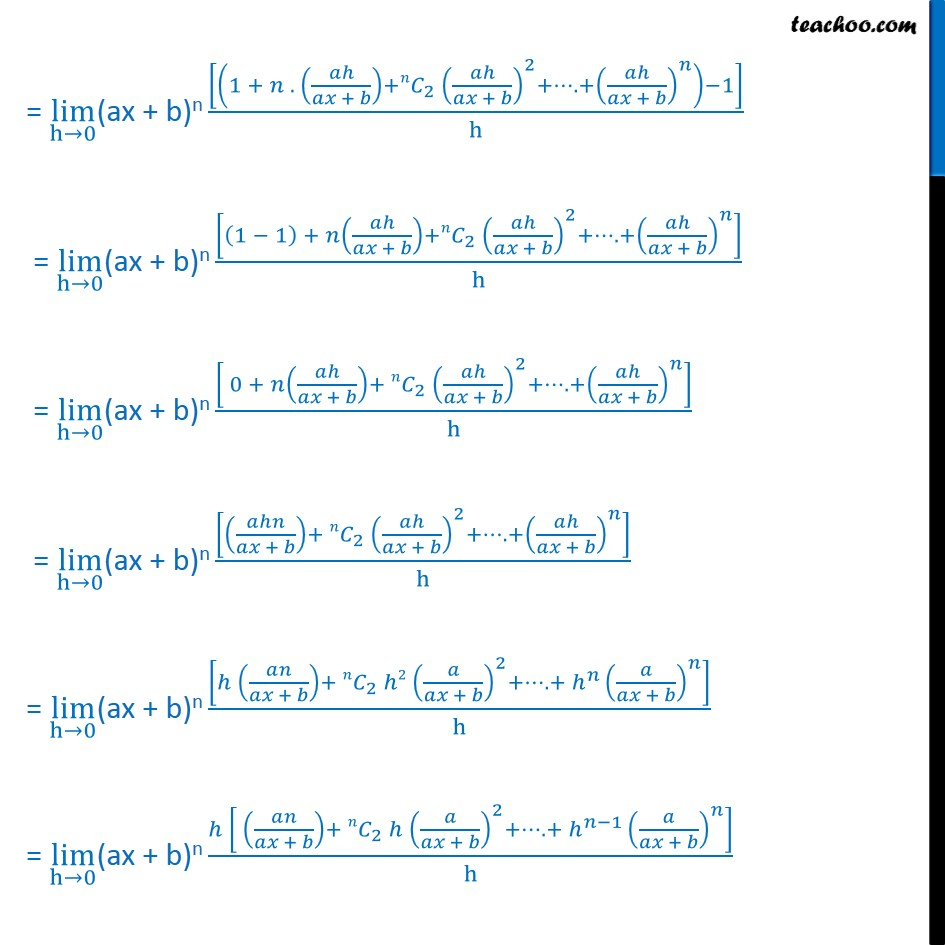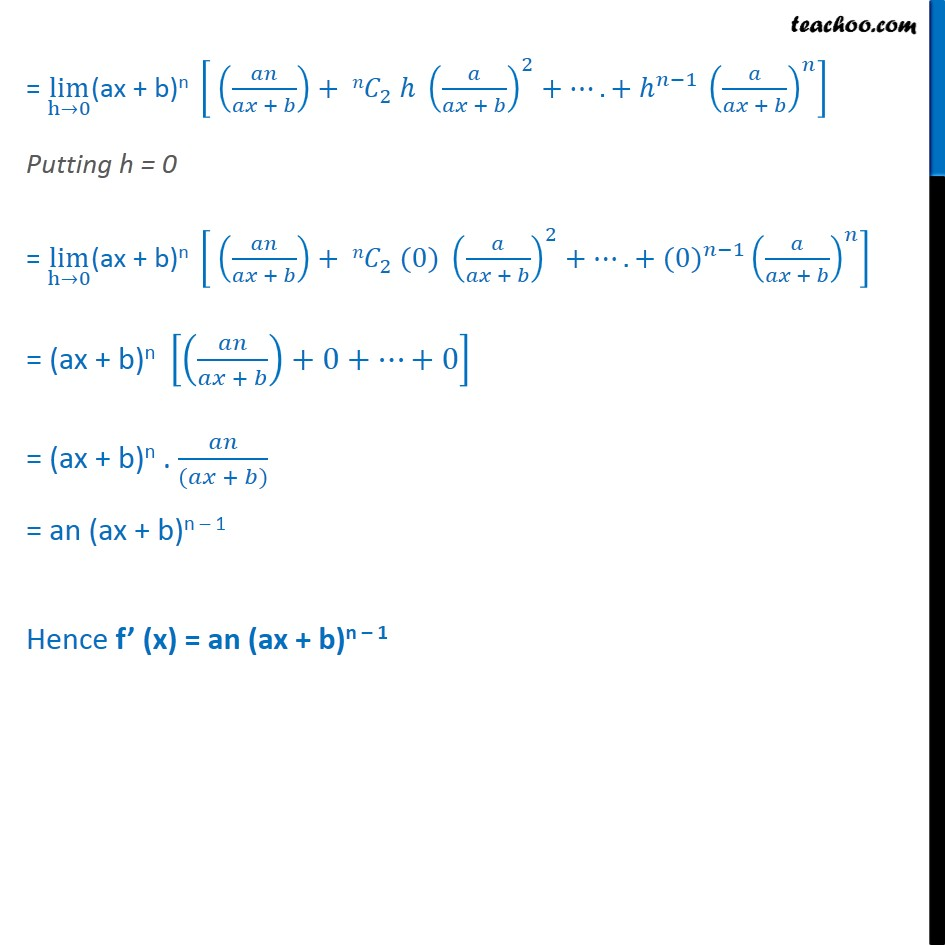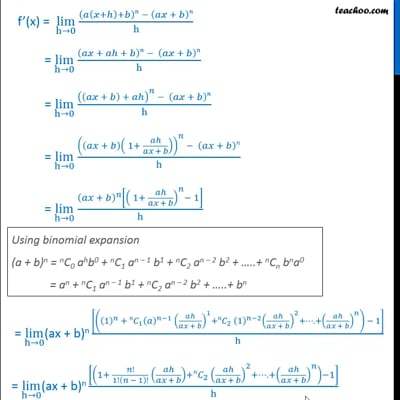This video is only available for Teachoo black users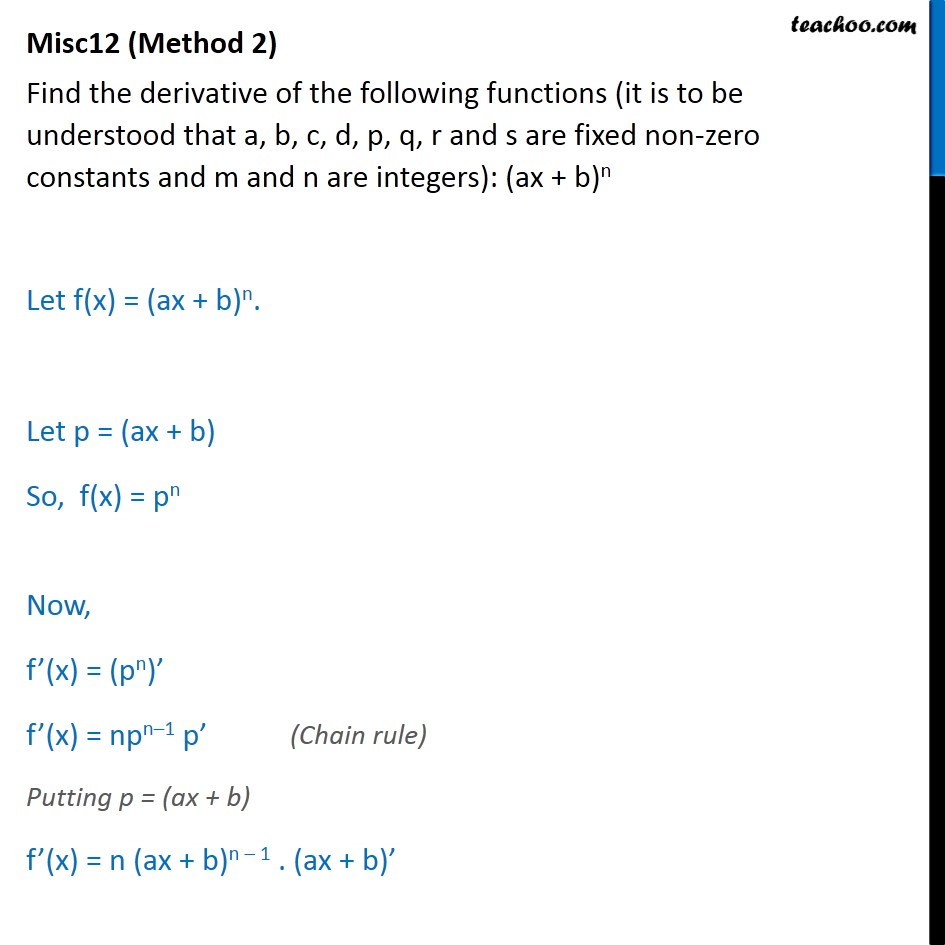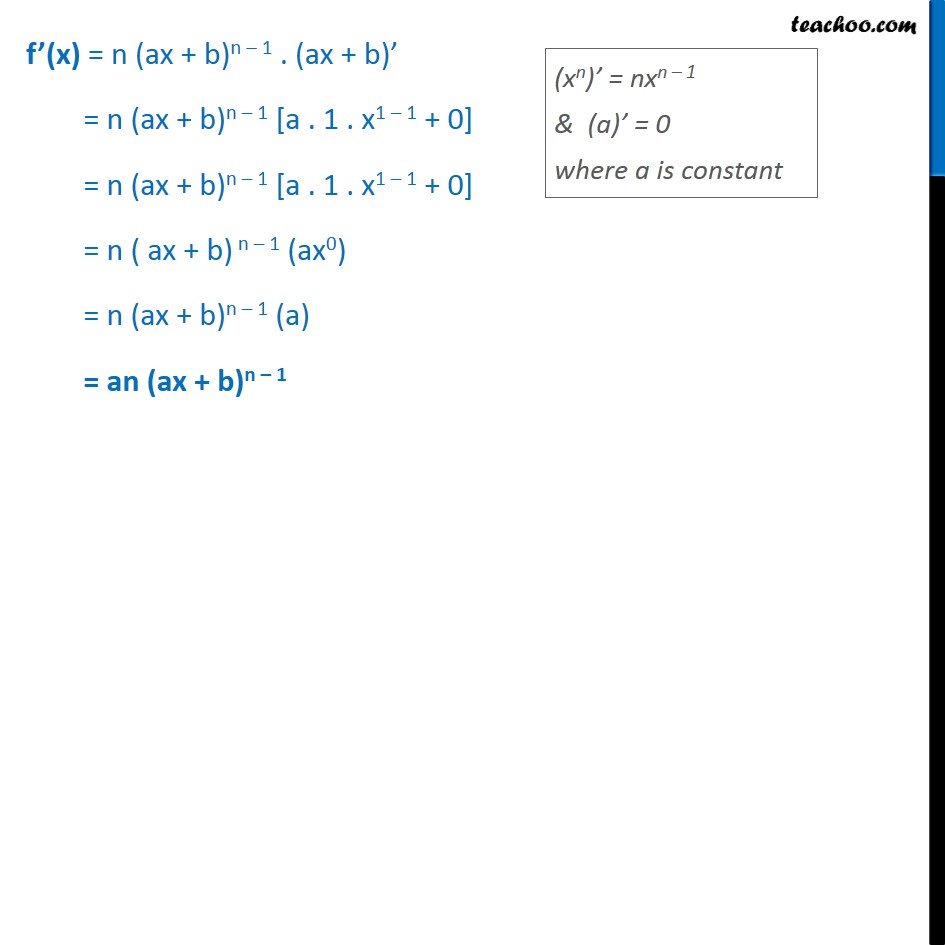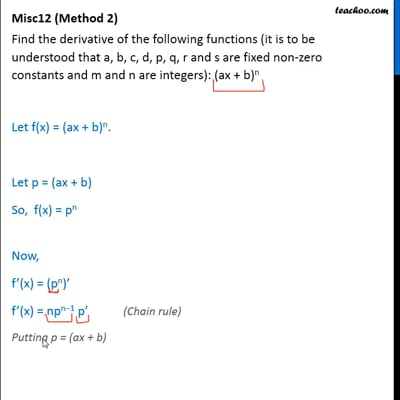This video is only available for Teachoo black users

### Transcript

Misc 12 (Method 1) Find the derivative of the following functions (it is to be understood that a, b, c, d, p, q, r and s are fixed non-zero constants and m and n are integers): (ax + b)n Let f(x) = (ax + b)n. We know that f’(x) = lim﷮h→0﷯ f x + h﷯ − f(x)﷮h﷯ Here, f(x) = (ax + b)n So, f(x + h) = (a(x + h) + b)n Putting values f’(x) = lim﷮h→0﷯ 𝑎 𝑥+ℎ﷯+𝑏﷯𝑛 − 𝑎𝑥 + 𝑏﷯𝑛﷮h﷯ f’(x) = lim﷮h→0﷯ 𝑎 𝑥+ℎ﷯+𝑏﷯𝑛 − 𝑎𝑥 + 𝑏﷯𝑛﷮h﷯ = lim﷮h→0﷯ 𝑎𝑥 + 𝑎ℎ + 𝑏﷯𝑛 − 𝑎𝑥 + 𝑏﷯𝑛﷮h﷯ = lim﷮h→0﷯ 𝑎𝑥 + 𝑏﷯ + 𝑎ℎ﷯﷮𝑛﷯ − 𝑎𝑥 + 𝑏﷯𝑛﷮h﷯ = lim﷮h→0﷯ 𝑎𝑥 + 𝑏﷯ 1+ 𝑎ℎ﷮𝑎𝑥 + 𝑏﷯﷯﷯﷮𝑛﷯ − 𝑎𝑥 + 𝑏﷯𝑛﷮h﷯ = lim﷮h→0﷯ 𝑎𝑥 + 𝑏﷯﷮𝑛﷯ 𝟏+ 𝒂𝒉﷮𝒂𝒙 + 𝒃﷯﷯﷮𝒏﷯− 1﷯﷮h﷯ = lim﷮h→0﷯(ax + b)n 𝟏﷯﷮𝒏﷯ + 𝒏𝑪﷮𝟏﷯ 𝒂﷯﷮𝒏−𝟏﷯ 𝒂𝒉﷮𝒂𝒙 + 𝒃﷯﷯﷮𝟏﷯+ 𝒏𝑪﷮𝟐﷯ 𝟏﷯﷮𝒏−𝟐﷯ 𝒂𝒉﷮𝒂𝒙 + 𝒃﷯﷯﷮𝟐﷯+…. + 𝒂𝒉﷮𝒂𝒙 + 𝒃﷯﷯﷮𝒏﷯﷯ − 1﷯﷮h﷯ = lim﷮h→0﷯(ax + b)n 1+ 𝑛!﷮1! 𝑛 − 1﷯!﷯ 𝑎ℎ﷮𝑎𝑥 + 𝑏﷯﷯+ 𝑛𝐶﷮2﷯ 𝑎ℎ﷮𝑎𝑥 + 𝑏﷯﷯﷮2﷯+…. + 𝑎ℎ﷮𝑎𝑥 + 𝑏﷯﷯﷮𝑛﷯﷯−1﷯﷮h﷯ = lim﷮h→0﷯(ax + b)n 1 + 𝑛 . 𝑎ℎ﷮𝑎𝑥 + 𝑏﷯﷯+ 𝑛𝐶﷮2﷯ 𝑎ℎ﷮𝑎𝑥 + 𝑏﷯﷯﷮2﷯+…. + 𝑎ℎ﷮𝑎𝑥 + 𝑏﷯﷯﷮𝑛﷯﷯−1﷯﷮h﷯ = lim﷮h→0﷯(ax + b)n 1 − 1﷯ + 𝑛 𝑎ℎ﷮𝑎𝑥 + 𝑏﷯﷯+ 𝑛𝐶﷮2﷯ 𝑎ℎ﷮𝑎𝑥 + 𝑏﷯﷯﷮2﷯+…. + 𝑎ℎ﷮𝑎𝑥 + 𝑏﷯﷯﷮𝑛﷯﷯﷮h﷯ = lim﷮h→0﷯(ax + b)n 0 + 𝑛 𝑎ℎ﷮𝑎𝑥 + 𝑏﷯﷯+ 𝑛𝐶﷮2﷯ 𝑎ℎ﷮𝑎𝑥 + 𝑏﷯﷯﷮2﷯+…. + 𝑎ℎ﷮𝑎𝑥 + 𝑏﷯﷯﷮𝑛﷯﷯﷮h﷯ = lim﷮h→0﷯(ax + b)n 𝑎ℎ𝑛﷮𝑎𝑥 + 𝑏﷯﷯+ 𝑛𝐶﷮2﷯ 𝑎ℎ﷮𝑎𝑥 + 𝑏﷯﷯﷮2﷯+…. + 𝑎ℎ﷮𝑎𝑥 + 𝑏﷯﷯﷮𝑛﷯﷯﷮h﷯ = lim﷮h→0﷯(ax + b)n ℎ 𝑎𝑛﷮𝑎𝑥 + 𝑏﷯﷯+ 𝑛𝐶﷮2﷯ ℎ2 𝑎﷮𝑎𝑥 + 𝑏﷯﷯﷮2﷯+…. + ℎ﷮𝑛﷯ 𝑎﷮𝑎𝑥 + 𝑏﷯﷯﷮𝑛﷯﷯﷮h﷯ = lim﷮h→0﷯(ax + b)n ℎ 𝑎𝑛﷮𝑎𝑥 + 𝑏﷯﷯+ 𝑛𝐶﷮2﷯ ℎ 𝑎﷮𝑎𝑥 + 𝑏﷯﷯﷮2﷯+…. + ℎ﷮𝑛−1﷯ 𝑎﷮𝑎𝑥 + 𝑏﷯﷯﷮𝑛﷯﷯﷮h﷯ = lim﷮h→0﷯(ax + b)n 𝑎𝑛﷮𝑎𝑥 + 𝑏﷯﷯+ 𝑛𝐶﷮2﷯ ℎ 𝑎﷮𝑎𝑥 + 𝑏﷯﷯﷮2﷯+…. + ℎ﷮𝑛−1﷯ 𝑎﷮𝑎𝑥 + 𝑏﷯﷯﷮𝑛﷯﷯ Putting h = 0 = lim﷮h→0﷯(ax + b)n 𝑎𝑛﷮𝑎𝑥 + 𝑏﷯﷯+ 𝑛𝐶﷮2﷯ (0) 𝑎﷮𝑎𝑥 + 𝑏﷯﷯﷮2﷯+…. + (0)﷮𝑛−1﷯ 𝑎﷮𝑎𝑥 + 𝑏﷯﷯﷮𝑛﷯﷯ = (ax + b)n 𝑎𝑛﷮𝑎𝑥 + 𝑏﷯﷯+0+…+0﷯ = (ax + b)n . 𝑎𝑛﷮(𝑎𝑥 + 𝑏)﷯ = an (ax + b)n – 1 Hence f’ (x) = an (ax + b)n – 1 Misc12 (Method 2) Find the derivative of the following functions (it is to be understood that a, b, c, d, p, q, r and s are fixed non-zero constants and m and n are integers): (ax + b)n Let f(x) = (ax + b)n. Let p = (ax + b) So, f(x) = pn Now, f’(x) = (pn)’ f’(x) = npn–1 p’ Putting p = (ax + b) f’(x) = n (ax + b)n – 1 . (ax + b)’ f’(x) = n (ax + b)n – 1 . (ax + b)’ = n (ax + b)n – 1 [a . 1 . x1 – 1 + 0] = n (ax + b)n – 1 [a . 1 . x1 – 1 + 0] = n ( ax + b) n – 1 (ax0) = n (ax + b)n – 1 (a) = an (ax + b)n – 1